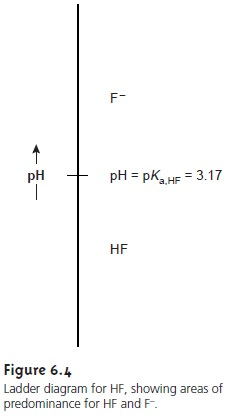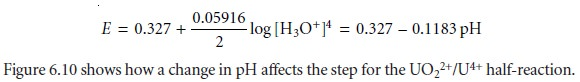Home | | Modern Analytical Chemistry | Ladder Diagrams

## Chapter: Modern Analytical Chemistry: Equilibrium Chemistry

1. Ladder Diagrams for Acidâ€“Base Equilibria 2. Ladder Diagrams for Complexation Equilibria 3. Ladder Diagrams for Oxidationâ€“Reduction Equilibria

When developing or evaluating an analytical method, we often need to under- stand how the chemistry taking place affects our results. We have already seen, for example, that adding NH3 to a solution of Ag+ is a poor idea if we intend to isolate the Ag+ as a precipitate of AgCl (reaction 6.29). One of the primary sources of determinate method errors is a failure to account for potential chemi- cal interferences.

In this section we introduce the ladder diagram as a simple graphical tool for evaluating the chemistry taking place during an analysis. Using ladder dia- grams, we will be able to determine what reactions occur when several reagents are combined, estimate the approximate composition of a system at equilibrium, and evaluate how a change in solution conditions might affect our results.

## Ladder Diagrams for Acidâ€“Base Equilibria

To see how a ladder diagram is constructed, we will use the acidâ€“base equilibrium between HF and Fâ€“Finally, replacing the negative log terms with p-functions and rearranging leaves us withExamining equation 6.31 tells us a great deal about the relationship between pH and the relative amounts of Fâ€“ and HF at equilibrium. If the concentrations of Fâ€“ and HF are equal, then equation 6.31 reduces to

pH= pKa,HF = â€“log(Ka,HF) = â€“log(6.8 x 10â€“4) = 3.17

For concentrations of Fâ€“ greater than that of HF, the log term in equation 6.31 is positive and

pH> pKa,HF or pH > 3.17

This is a reasonable result since we expect the concentration of hydrofluoric acidâ€™s conjugate base, Fâ€“, to increase as the pH increases. Similar reasoning shows that the concentration of HF exceeds that of Fâ€“ when

pH < pKa,HF or pH < 3.17

Now we are ready to construct the ladder diagram for HF (Figure 6.4). The ladder diagram consists of a vertical scale of pH values oriented so that smaller (more acidic) pH levels are at the bottom and larger (more basic) pH levels are at the top. A horizontal line is drawn at a pH equal to pKa,HF. This line, or step, separates the solution into regions where each of the two conjugate forms of HF predominate.By referring to the ladder diagram, we see that at a pH of 2.5 hydrofluoric acid will exist predominately as HF. If we add sufficient base to the solution such that the pH increases to 4.5, the predominate form be- comes Fâ€“.

Figure 6.5 shows a second ladder diagram containing information about  HF/Fâ€“ and NH4 +/NH3 .  From this ladder diagram we see that if the pH is less than 3.17, the predominate species are HF and NH4+. For pHâ€™s between 3.17 and 9.24 the predominate species are Fâ€“ and NH4+, whereas above a pH of 9.24 the predominate species are Fâ€“ and NH3.Ladder diagrams are particularly useful for evaluating the reactivity of acids and bases. An acid and a base cannot coexist if their respective areas of predominance do not overlap. If we mix together solutions of NH3 and HF, the reactionoccurs because the predominance areas for HF and NH3 do not overlap. Be- fore continuing, let us show that this conclusion is reasonable by calculating the equilibrium constant for reaction 6.32. To do so we need the following three reactions and their equilibrium constants.Since the equilibrium constant is significantly greater than 1, the reactionâ€™s equilib- rium position lies far to the right. This conclusion is general and applies to all lad- der diagrams. The following example shows how we can use the ladder diagram in Figure 6.5 to evaluate the composition of any solution prepared by mixing together solutions of HF and NH3.If the areas of predominance for an acid and a base overlap each other, then practically no reaction occurs. For example, if we mix together solutions of NaF and NH4Cl, we expect that there will be no significant change in the moles of Fâ€“ and NH4+. Furthermore, the pH of the mixture must be between 3.17 and 9.24. Because Fâ€“ and NH4+ can coexist over a range of pHs we cannot be more specific in estimat- ing the solutionâ€™s pH.

The ladder diagram for HF/Fâ€“ also can be used to evaluate the effect of pH on other equilibria that include either HF or Fâ€“. For example, the solubility of CaF2

CaF2(s)  < = = = >  tCa2+(aq)+ 2Fâ€“(aq)

is affected by pH because Fâ€“ is a weak base. Using Le ChĂ˘telierâ€™s principle, if Fâ€“ is converted to HF, the solubility of CaF2 will increase. To minimize the solubility of CaF2 we want to control the solutionâ€™s pH so that Fâ€“ is the predominate species. From the ladder diagram we see that maintaining a pH of more than 3.17 ensures that solubility losses are minimal.

## Ladder Diagrams for Complexation Equilibria

The same principles used in constructing and interpreting ladder diagrams for acidâ€“base equilibria can be applied to equilibria involving metalâ€“ligand com- plexes. For complexation reactions the ladder diagramâ€™s scale is defined by the concentration of uncomplexed, or free ligand, pL. Using the formation of Cd(NH3)2+ as an examplewe can easily show that the dividing line between the predominance regions for Cd2+ and Cd(NH3)2+ is log(K1).Since K1 for Cd(NH3)2+ is 3.55 Â´ 102, log(K1) is 2.55. Thus, for a pNH3 greater than 2.55 (concentrations of NH3 less than 2.8 x 10â€“3 M), Cd2+ is the predominate species. A complete ladder diagram for the metalâ€“ligand complexes of Cd2+ and NH3 is shown in Figure 6.6.We can also construct ladder diagrams using cumulative formation constants in place of stepwise formation constants. The first three stepwise formation con- stants for the reaction of Zn2+ with NH3show that the formation of Zn(NH3)32+ is more favorable than the formation of Zn(NH3)2+ or Zn(NH3)22+. The equilibrium, therefore, is best represented by the cumulative formation reactionA complete ladder diagram for the Zn2+â€“NH3 system is shown in Figure 6.8.## Ladder Diagram for Oxidationâ€“Reduction Equilibria

Ladder diagrams can also be used to evaluate equilibrium reactions in redox sys- tems. Figure 6.9 shows a typical ladder diagram for two half-reactions in which the scale is the electrochemical potential, E. Areas of predominance are defined by the Nernst equation. Using the Fe3+/Fe2+ half-reaction as an example, we writeFor potentials more positive than the standard-state potential, the predominate species is Fe3+, whereas Fe2+ predominates for potentials more negative than EÂ°. When coupled with the step for the Sn4+/Sn2+ half-reaction, we see that Sn2+ can be used to reduce Fe3+. If an excess of Sn2+ is added, the potential of the resulting solu- tion will be near +0.154 V.

Using standard-state potentials to construct a ladder diagram can present problems if solutes are not at their standard-state concentra- tions. Because the concentrations of the reduced and oxidized species are in a logarithmic term, deviations from standard-state concentra- tions can usually be ignored if the steps being compared are separated by at least 0.3 V.1b A trickier problem occurs when a half-reactionâ€™s po- tential is affected by the concentration of another species. For example, the potential for the following half-reactiondepends on the pH of the solution. To define areas of predominance in this case, we begin with the Nernst equationand factor out the concentration of H3O+.From this equation we see that the areas of predominance for UO22+ and U4+ are defined by a step whose potential isStudy Material, Lecturing Notes, Assignment, Reference, Wiki description explanation, brief detail
Modern Analytical Chemistry: Equilibrium Chemistry : Ladder Diagrams |